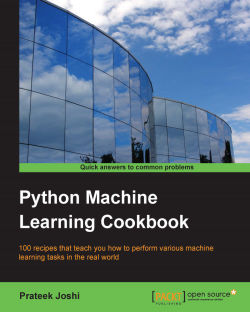# Python Machine Learning Cookbook4.4 (8 reviews total)
By Prateek Joshi
• FREE Subscription Access now
• \$12.99 eBook + Subscription
What do you get with a Packt Subscription?

• Constantly updated with 100+ new titles each month
• Breadth and depth in over 1,000+ technologies

Machine learning is becoming increasingly pervasive in the modern data-driven world. It is used extensively across many fields such as search engines, robotics, self-driving cars, and more.

With this book, you will learn how to perform various machine learning tasks in different environments. We’ll start by exploring a range of real-life scenarios where machine learning can be used, and look at various building blocks. Throughout the book, you’ll use a wide variety of machine learning algorithms to solve real-world problems and use Python to implement these algorithms.

You’ll discover how to deal with various types of data and explore the differences between machine learning paradigms such as supervised and unsupervised learning. We also cover a range of regression techniques, classification algorithms, predictive modeling, data visualization techniques, recommendation engines, and more with the help of real-world examples.

Publication date:
June 2016
Publisher
Packt
Pages
304
ISBN
9781786464477

## Chapter 1. The Realm of Supervised Learning

In this chapter, we will cover the following recipes:

• Preprocessing data using different techniques

• Label encoding

• Building a linear regressor

• Computing regression accuracy

• Achieving model persistence

• Building a ridge regressor

• Building a polynomial regressor

• Estimating housing prices

• Computing the relative importance of features

• Estimating bicycle demand distribution

## Introduction

If you are familiar with the basics of machine learning, you will certainly know what supervised learning is all about. To give you a quick refresher, supervised learning refers to building a machine learning model that is based on labeled samples. For example, if we build a system to estimate the price of a house based on various parameters, such as size, locality, and so on, we first need to create a database and label it. We need to tell our algorithm what parameters correspond to what prices. Based on this data, our algorithm will learn how to calculate the price of a house using the input parameters.

Unsupervised learning is the opposite of what we just discussed. There is no labeled data available here. Let's assume that we have a bunch of datapoints, and we just want to separate them into multiple groups. We don't exactly know what the criteria of separation would be. So, an unsupervised learning algorithm will try to separate the given dataset into a fixed number of groups in the best possible way. We will discuss unsupervised learning in the upcoming chapters.

We will use various Python packages, such as NumPy, SciPy, scikit-learn, and matplotlib, during the course of this book to build various things. If you use Windows, it is recommended that you use a SciPy-stack compatible version of Python. You can check the list of compatible versions at http://www.scipy.org/install.html. These distributions come with all the necessary packages already installed. If you use Mac OS X or Ubuntu, installing these packages is fairly straightforward. Here are some useful links for installation and documentation:

Make sure that you have these packages installed on your machine before you proceed.

## Preprocessing data using different techniques

In the real world, we usually have to deal with a lot of raw data. This raw data is not readily ingestible by machine learning algorithms. To prepare the data for machine learning, we have to preprocess it before we feed it into various algorithms.

Let's see how to preprocess data in Python. To start off, open a file with a `.py` extension, for example, `preprocessor.py`, in your favorite text editor. Add the following lines to this file:

```import numpy as np
from sklearn import preprocessing```

We just imported a couple of necessary packages. Let's create some sample data. Add the following line to this file:

`data = np.array([[3, -1.5,  2, -5.4], [0,  4,  -0.3, 2.1], [1,  3.3, -1.9, -4.3]])`

We are now ready to operate on this data.

### How to do it…

Data can be preprocessed in many ways. We will discuss a few of the most commonly-used preprocessing techniques.

#### Mean removal

It's usually beneficial to remove the mean from each feature so that it's centered on zero. This helps us in removing any bias from the features. Add the following lines to the file that we opened earlier:

```data_standardized = preprocessing.scale(data)
print "\nMean =", data_standardized.mean(axis=0)
print "Std deviation =", data_standardized.std(axis=0)```

We are now ready to run the code. To do this, run the following command on your Terminal:

```\$ python preprocessor.py
```

You will see the following output on your Terminal:

```Mean = [  5.55111512e-17  -1.11022302e-16  -7.40148683e-17  -7.40148683e-17]
Std deviation = [ 1.  1.  1.  1.]
```

You can see that the mean is almost `0` and the standard deviation is `1`.

#### Scaling

The values of each feature in a datapoint can vary between random values. So, sometimes it is important to scale them so that this becomes a level playing field. Add the following lines to the file and run the code:

```data_scaler = preprocessing.MinMaxScaler(feature_range=(0, 1))
data_scaled = data_scaler.fit_transform(data)
print "\nMin max scaled data =", data_scaled```

After scaling, all the feature values range between the specified values. The output will be displayed, as follows:

```Min max scaled data:
[[ 1.          0.          1.          0.        ]
[ 0.          1.          0.41025641  1.        ]
[ 0.33333333  0.87272727  0.          0.14666667]]
```

#### Normalization

Data normalization is used when you want to adjust the values in the feature vector so that they can be measured on a common scale. One of the most common forms of normalization that is used in machine learning adjusts the values of a feature vector so that they sum up to 1. Add the following lines to the previous file:

```data_normalized = preprocessing.normalize(data, norm='l1')
print "\nL1 normalized data =", data_normalized```

If you run the Python file, you will get the following output:

```L1 normalized data:
[[ 0.25210084 -0.12605042  0.16806723 -0.45378151]
[ 0.          0.625      -0.046875    0.328125  ]
[ 0.0952381   0.31428571 -0.18095238 -0.40952381]]
```

This is used a lot to make sure that datapoints don't get boosted artificially due to the fundamental nature of their features.

#### Binarization

Binarization is used when you want to convert your numerical feature vector into a Boolean vector. Add the following lines to the Python file:

```data_binarized = preprocessing.Binarizer(threshold=1.4).transform(data)
print "\nBinarized data =", data_binarized```

Run the code again, and you will see the following output:

```Binarized data:
[[ 1.  0.  1.  0.]
[ 0.  1.  0.  1.]
[ 0.  1.  0.  0.]]
```

This is a very useful technique that's usually used when we have some prior knowledge of the data.

#### One Hot Encoding

A lot of times, we deal with numerical values that are sparse and scattered all over the place. We don't really need to store these big values. This is where One Hot Encoding comes into picture. We can think of One Hot Encoding as a tool to tighten the feature vector. It looks at each feature and identifies the total number of distinct values. It uses a one-of-k scheme to encode the values. Each feature in the feature vector is encoded based on this. This helps us be more efficient in terms of space. For example, let's say we are dealing with 4-dimensional feature vectors. To encode the n-th feature in a feature vector, the encoder will go through the n-th feature in each feature vector and count the number of distinct values. If the number of distinct values is k, it will transform the feature into a k-dimensional vector where only one value is 1 and all other values are 0. Add the following lines to the Python file:

```encoder = preprocessing.OneHotEncoder()
encoder.fit([[0, 2, 1, 12], [1, 3, 5, 3], [2, 3, 2, 12], [1, 2, 4, 3]])
encoded_vector = encoder.transform([[2, 3, 5, 3]]).toarray()
print "\nEncoded vector =", encoded_vector```

This is the expected output:

```Encoded vector:
[[ 0.  0.  1.  0.  1.  0.  0.  0.  1.  1.  0.]]
```

In the above example, let's consider the third feature in each feature vector. The values are `1`, `5`, `2`, and `4`. There are four distinct values here, which means the one-hot encoded vector will be of length 4. If you want to encode the value `5`, it will be a vector `[0, 1, 0, 0]`. Only one value can be 1 in this vector. The second element is 1, which indicates that the value is `5`.

## Label encoding

In supervised learning, we usually deal with a variety of labels. These can be in the form of numbers or words. If they are numbers, then the algorithm can use them directly. However, a lot of times, labels need to be in human readable form. So, people usually label the training data with words. Label encoding refers to transforming the word labels into numerical form so that the algorithms can understand how to operate on them. Let's take a look at how to do this.

### How to do it…

1. Create a new Python file, and import the preprocessing package:

`from sklearn import preprocessing`
2. This package contains various functions that are needed for data preprocessing. Let's define the label encoder, as follows:

`label_encoder = preprocessing.LabelEncoder()`
3. The `label_encoder` object knows how to understand word labels. Let's create some labels:

`input_classes = ['audi', 'ford', 'audi', 'toyota', 'ford', 'bmw']`
4. We are now ready to encode these labels:

```label_encoder.fit(input_classes)
print "\nClass mapping:"
for i, item in enumerate(label_encoder.classes_):
print item, '-->', i```
5. Run the code, and you will see the following output on your Terminal:

```Class mapping:
audi --> 0
bmw --> 1
ford --> 2
toyota --> 3
```
6. As shown in the preceding output, the words have been transformed into 0-indexed numbers. Now, when you encounter a set of labels, you can simply transform them, as follows:

```labels = ['toyota', 'ford', 'audi']
encoded_labels = label_encoder.transform(labels)
print "\nLabels =", labels
print "Encoded labels =", list(encoded_labels)```

Here is the output that you'll see on your Terminal:

```Labels = ['toyota', 'ford', 'audi']
Encoded labels = [3, 2, 0]
```
7. This is way easier than manually maintaining mapping between words and numbers. You can check the correctness by transforming numbers back to word labels:

```encoded_labels = [2, 1, 0, 3, 1]
decoded_labels = label_encoder.inverse_transform(encoded_labels)
print "\nEncoded labels =", encoded_labels
print "Decoded labels =", list(decoded_labels)```

Here is the output:

```Encoded labels = [2, 1, 0, 3, 1]
Decoded labels = ['ford', 'bmw', 'audi', 'toyota', 'bmw']
```

As you can see, the mapping is preserved perfectly.

## Building a linear regressor

Regression is the process of estimating the relationship between input data and the continuous-valued output data. This data is usually in the form of real numbers, and our goal is to estimate the underlying function that governs the mapping from the input to the output. Let's start with a very simple example. Consider the following mapping between input and output:

1 --> 2

3 --> 6

4.3 --> 8.6

7.1 --> 14.2

If I ask you to estimate the relationship between the inputs and the outputs, you can easily do this by analyzing the pattern. We can see that the output is twice the input value in each case, so the transformation would be as follows:

f(x) = 2x

This is a simple function, relating the input values with the output values. However, in the real world, this is usually not the case. Functions in the real world are not so straightforward!

Linear regression refers to estimating the underlying function using a linear combination of input variables. The preceding example was an example that consisted of one input variable and one output variable.

Consider the following figure: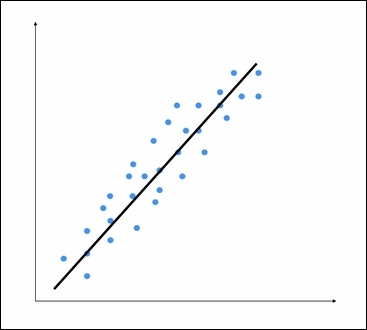The goal of linear regression is to extract the underlying linear model that relates the input variable to the output variable. This aims to minimize the sum of squares of differences between the actual output and the predicted output using a linear function. This method is called Ordinary least squares.

You might say that there might be a curvy line out there that fits these points better, but linear regression doesn't allow this. The main advantage of linear regression is that it's not complex. If you go into nonlinear regression, you may get more accurate models, but they will be slower. As shown in the preceding figure, the model tries to approximate the input datapoints using a straight line. Let's see how to build a linear regression model in Python.

### How to do it…

You have been provided with a data file, called `data_singlevar.txt`. This contains comma-separated lines where the first element is the input value and the second element is the output value that corresponds to this input value. You should use this as the input argument:

1. Create a file called `regressor.py`, and add the following lines:

```import sys
import numpy as np
filename = sys.argv
X = []
y = []
with open(filename, 'r') as f:
xt, yt = [float(i) for i in line.split(',')]
X.append(xt)
y.append(yt)```

We just loaded the input data into `X` and `y`, where `X` refers to data and `y` refers to labels. Inside the loop in the preceding code, we parse each line and split it based on the comma operator. We then convert it into floating point values and save it in `X` and `y`, respectively.

2. When we build a machine learning model, we need a way to validate our model and check whether the model is performing at a satisfactory level. To do this, we need to separate our data into two groups: a training dataset and a testing dataset. The training dataset will be used to build the model, and the testing dataset will be used to see how this trained model performs on unknown data. So, let's go ahead and split this data into training and testing datasets:

```num_training = int(0.8 * len(X))
num_test = len(X) - num_training

# Training data
X_train = np.array(X[:num_training]).reshape((num_training,1))
y_train = np.array(y[:num_training])

# Test data
X_test = np.array(X[num_training:]).reshape((num_test,1))
y_test = np.array(y[num_training:])```

Here, we will use 80% of the data for the training dataset and the remaining 20% for the testing dataset.

3. We are now ready to train the model. Let's create a regressor object, as follows:

```from sklearn import linear_model

# Create linear regression object
linear_regressor = linear_model.LinearRegression()

# Train the model using the training sets
linear_regressor.fit(X_train, y_train)```
4. We just trained the linear regressor, based on our training data. The fit method takes the input data and trains the model. Let's see how it fits:

```import matplotlib.pyplot as plt

y_train_pred = linear_regressor.predict(X_train)
plt.figure()
plt.scatter(X_train, y_train, color='green')
plt.plot(X_train, y_train_pred, color='black', linewidth=4)
plt.title('Training data')
plt.show()```
5. We are now ready to run the code using the following command:

```\$ python regressor.py data_singlevar.txt
```

You should see the following figure: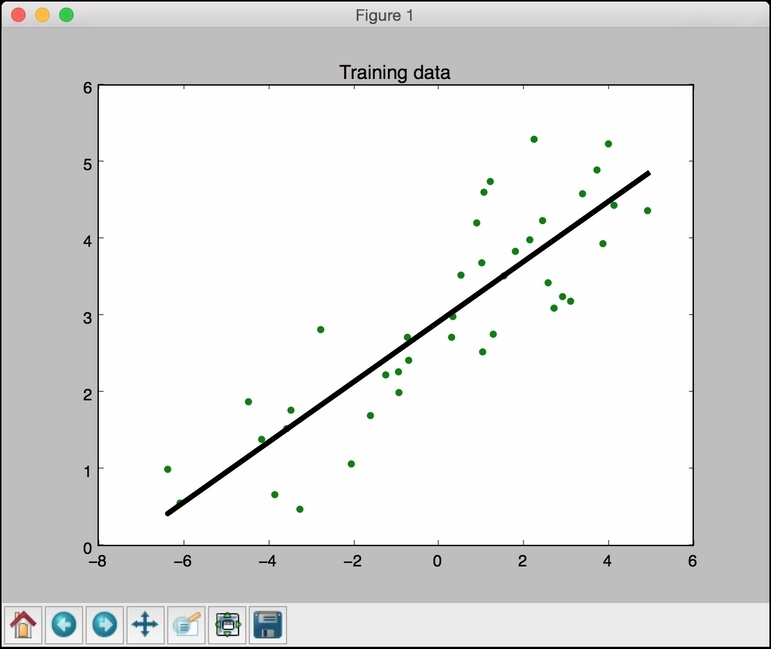6. In the preceding code, we used the trained model to predict the output for our training data. This wouldn't tell us how the model performs on unknown data because we are running it on training data itself. This just gives us an idea of how the model fits on training data. Looks like it's doing okay as you can see in the preceding figure!

7. Let's predict the test dataset output based on this model and plot it, as follows:

```y_test_pred = linear_regressor.predict(X_test)

plt.scatter(X_test, y_test, color='green')
plt.plot(X_test, y_test_pred, color='black', linewidth=4)
plt.title('Test data')
plt.show()```

If you run this code, you will see a graph like the following one: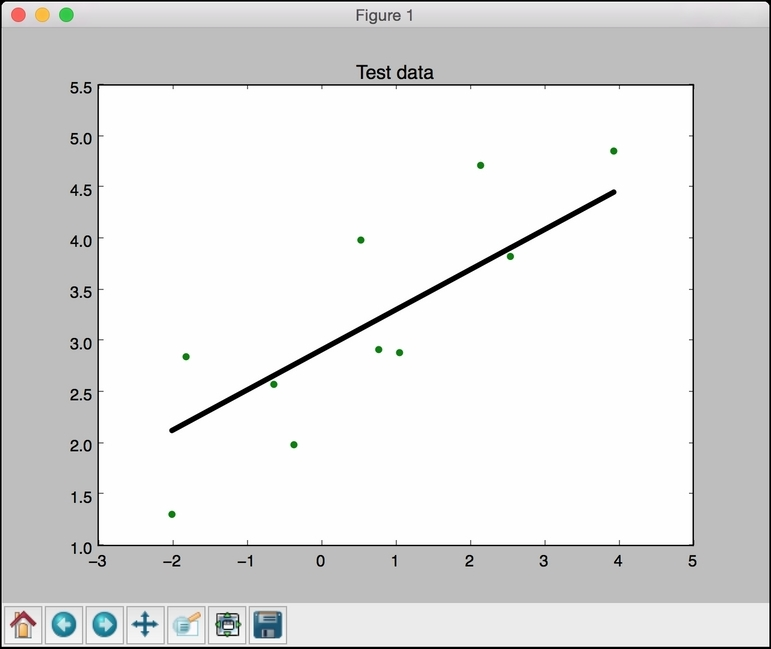## Computing regression accuracy

Now that we know how to build a regressor, it's important to understand how to evaluate the quality of a regressor as well. In this context, an error is defined as the difference between the actual value and the value that is predicted by the regressor.

Let's quickly understand what metrics can be used to measure the quality of a regressor. A regressor can be evaluated using many different metrics, such as the following:

• Mean absolute error: This is the average of absolute errors of all the datapoints in the given dataset.

• Mean squared error: This is the average of the squares of the errors of all the datapoints in the given dataset. It is one of the most popular metrics out there!

• Median absolute error: This is the median of all the errors in the given dataset. The main advantage of this metric is that it's robust to outliers. A single bad point in the test dataset wouldn't skew the entire error metric, as opposed to a mean error metric.

• Explained variance score: This score measures how well our model can account for the variation in our dataset. A score of 1.0 indicates that our model is perfect.

• R2 score: This is pronounced as R-squared, and this score refers to the coefficient of determination. This tells us how well the unknown samples will be predicted by our model. The best possible score is 1.0, and the values can be negative as well.

### How to do it…

There is a module in scikit-learn that provides functionalities to compute all the following metrics. Open a new Python file and add the following lines:

```import sklearn.metrics as sm

print "Mean absolute error =", round(sm.mean_absolute_error(y_test, y_test_pred), 2)
print "Mean squared error =", round(sm.mean_squared_error(y_test, y_test_pred), 2)
print "Median absolute error =", round(sm.median_absolute_error(y_test, y_test_pred), 2)
print "Explained variance score =", round(sm.explained_variance_score(y_test, y_test_pred), 2)
print "R2 score =", round(sm.r2_score(y_test, y_test_pred), 2)```

Keeping track of every single metric can get tedious, so we pick one or two metrics to evaluate our model. A good practice is to make sure that the mean squared error is low and the explained variance score is high.

## Achieving model persistence

When we train a model, it would be nice if we could save it as a file so that it can be used later by simply loading it again.

### How to do it…

Let's see how to achieve model persistence programmatically:

1. Add the following lines to `regressor.py`:

```import cPickle as pickle

output_model_file = 'saved_model.pkl'
with open(output_model_file, 'w') as f:
pickle.dump(linear_regressor, f)```
2. The regressor object will be saved in the `saved_model.pkl` file. Let's look at how to load it and use it, as follows:

```with open(output_model_file, 'r') as f:

y_test_pred_new = model_linregr.predict(X_test)
print "\nNew mean absolute error =", round(sm.mean_absolute_error(y_test, y_test_pred_new), 2)```
3. Here, we just loaded the regressor from the file into the `model_linregr` variable. You can compare the preceding result with the earlier result to confirm that it's the same.

## Building a ridge regressor

One of the main problems of linear regression is that it's sensitive to outliers. During data collection in the real world, it's quite common to wrongly measure the output. Linear regression uses ordinary least squares, which tries to minimize the squares of errors. The outliers tend to cause problems because they contribute a lot to the overall error. This tends to disrupt the entire model.

Let's consider the following figure: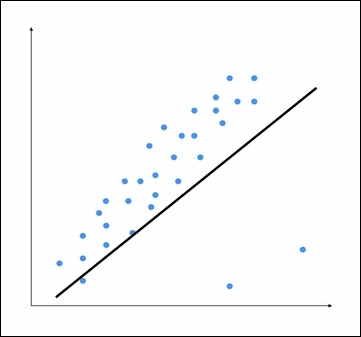The two points on the bottom are clearly outliers, but this model is trying to fit all the points. Hence, the overall model tends to be inaccurate. By visual inspection, we can see that the following figure is a better model: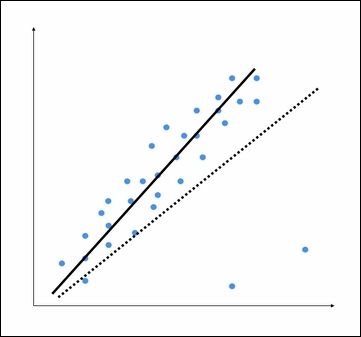Ordinary least squares considers every single datapoint when it's building the model. Hence, the actual model ends up looking like the dotted line as shown in the preceding figure. We can clearly see that this model is suboptimal. To avoid this, we use regularization where a penalty is imposed on the size of the coefficients. This method is called Ridge Regression.

### How to do it…

Let's see how to build a ridge regressor in Python:

1. You can load the data from the `data_multi_variable.txt` file. This file contains multiple values in each line. All the values except the last value form the input feature vector.

2. Add the following lines to `regressor.py`. Let's initialize a ridge regressor with some parameters:

`ridge_regressor = linear_model.Ridge(alpha=0.01, fit_intercept=True, max_iter=10000)`
3. The `alpha` parameter controls the complexity. As `alpha` gets closer to `0`, the ridge regressor tends to become more like a linear regressor with ordinary least squares. So, if you want to make it robust against outliers, you need to assign a higher value to `alpha`. We considered a value of `0.01`, which is moderate.

4. Let's train this regressor, as follows:

```ridge_regressor.fit(X_train, y_train)
y_test_pred_ridge = ridge_regressor.predict(X_test)
print "Mean absolute error =", round(sm.mean_absolute_error(y_test, y_test_pred_ridge), 2)
print "Mean squared error =", round(sm.mean_squared_error(y_test, y_test_pred_ridge), 2)
print "Median absolute error =", round(sm.median_absolute_error(y_test, y_test_pred_ridge), 2)
print "Explain variance score =", round(sm.explained_variance_score(y_test, y_test_pred_ridge), 2)
print "R2 score =", round(sm.r2_score(y_test, y_test_pred_ridge), 2)```

Run this code to view the error metrics. You can build a linear regressor to compare and contrast the results on the same data to see the effect of introducing regularization into the model.

## Building a polynomial regressor

One of the main constraints of a linear regression model is the fact that it tries to fit a linear function to the input data. The polynomial regression model overcomes this issue by allowing the function to be a polynomial, thereby increasing the accuracy of the model.

Let's consider the following figure: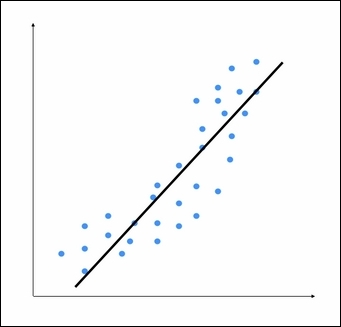We can see that there is a natural curve to the pattern of datapoints. This linear model is unable to capture this. Let's see what a polynomial model would look like: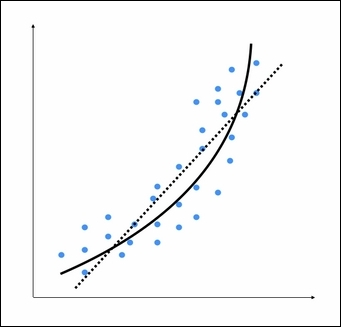The dotted line represents the linear regression model, and the solid line represents the polynomial regression model. The curviness of this model is controlled by the degree of the polynomial. As the curviness of the model increases, it gets more accurate. However, curviness adds complexity to the model as well, hence, making it slower. This is a trade off where you have to decide between how accurate you want your model to be given the computational constraints.

### How to do it…

1. Add the following lines to `regressor.py`:

```from sklearn.preprocessing import PolynomialFeatures

polynomial = PolynomialFeatures(degree=3)```
2. We initialized a polynomial of the degree `3` in the previous line. Now we have to represent the datapoints in terms of the coefficients of the polynomial:

```X_train_transformed = polynomial.fit_transform(X_train)
```

Here, `X_train_transformed` represents the same input in the polynomial form.

3. Let's consider the first datapoint in our file and check whether it can predict the right output:

```datapoint = [0.39,2.78,7.11]
poly_datapoint = polynomial.fit_transform(datapoint)

poly_linear_model = linear_model.LinearRegression()
poly_linear_model.fit(X_train_transformed, y_train)
print "\nLinear regression:", linear_regressor.predict(datapoint)
print "\nPolynomial regression:", poly_linear_model.predict(poly_datapoint)```

The values in the variable datapoint are the values in the first line in the input data file. We are still fitting a linear regression model here. The only difference is in the way in which we represent the data. If you run this code, you will see the following output:

```Linear regression: -11.0587294983
Polynomial regression: -10.9480782122
```

As you can see, this is close to the output value. If we want it to get closer, we need to increase the degree of the polynomial.

4. Let's make it `10` and see what happens:

`polynomial = PolynomialFeatures(degree=10)`

You should see something like the following:

```Polynomial regression: -8.20472183853
```

Now, you can see that the predicted value is much closer to the actual output value.

## Estimating housing prices

It's time to apply our knowledge to a real world problem. Let's apply all these principles to estimate the housing prices. This is one of the most popular examples that is used to understand regression, and it serves as a good entry point. This is intuitive and relatable, hence making it easier to understand concepts before we perform more complex things in machine learning. We will use a decision tree regressor with AdaBoost to solve this problem.

A decision tree is a tree where each node makes a simple decision that contributes to the final output. The leaf nodes represent the output values, and the branches represent the intermediate decisions that were made, based on input features. AdaBoost stands for Adaptive Boosting, and this is a technique that is used to boost the accuracy of the results from another system. This combines the outputs from different versions of the algorithms, called weak learners, using a weighted summation to get the final output. The information that's collected at each stage of the AdaBoost algorithm is fed back into the system so that the learners at the latter stages focus on training samples that are difficult to classify. This is the way it increases the accuracy of the system.

Using AdaBoost, we fit a regressor on the dataset. We compute the error and then fit the regressor on the same dataset again, based on this error estimate. We can think of this as fine-tuning of the regressor until the desired accuracy is achieved. You are given a dataset that contains various parameters that affect the price of a house. Our goal is to estimate the relationship between these parameters and the house price so that we can use this to estimate the price given unknown input parameters.

### How to do it…

1. Create a new file called `housing.py`, and add the following lines:

```import numpy as np
from sklearn.tree import DecisionTreeRegressor
from sklearn import datasets
from sklearn.metrics import mean_squared_error, explained_variance_score
from sklearn.utils import shuffle
import matplotlib.pyplot as plt```
2. There is a standard housing dataset that people tend to use to get started with machine learning. You can download it at https://archive.ics.uci.edu/ml/datasets/Housing. The good thing is that scikit-learn provides a function to directly load this dataset:

`housing_data = datasets.load_boston() `

Each datapoint has 13 input parameters that affect the price of the house. You can access the input data using `housing_data.data` and the corresponding price using `housing_data.target`.

3. Let's separate this into input and output. To make this independent of the ordering of the data, let's shuffle it as well:

`X, y = shuffle(housing_data.data, housing_data.target, random_state=7)`
4. The `random_state` parameter controls how we shuffle the data so that we can have reproducible results. Let's divide the data into training and testing. We'll allocate 80% for training and 20% for testing:

```num_training = int(0.8 * len(X))
X_train, y_train = X[:num_training], y[:num_training]
X_test, y_test = X[num_training:], y[num_training:]```
5. We are now ready to fit a decision tree regression model. Let's pick a tree with a maximum depth of `4`, which means that we are not letting the tree become arbitrarily deep:

```dt_regressor = DecisionTreeRegressor(max_depth=4)
dt_regressor.fit(X_train, y_train)```
6. Let's also fit decision tree regression model with AdaBoost:

```ab_regressor = AdaBoostRegressor(DecisionTreeRegressor(max_depth=4), n_estimators=400, random_state=7)
ab_regressor.fit(X_train, y_train)```

This will help us compare the results and see how AdaBoost really boosts the performance of a decision tree regressor.

7. Let's evaluate the performance of decision tree regressor:

```y_pred_dt = dt_regressor.predict(X_test)
mse = mean_squared_error(y_test, y_pred_dt)
evs = explained_variance_score(y_test, y_pred_dt)
print "\n#### Decision Tree performance ####"
print "Mean squared error =", round(mse, 2)
print "Explained variance score =", round(evs, 2)```
8. Now, let's evaluate the performance of AdaBoost:

```y_pred_ab = ab_regressor.predict(X_test)
mse = mean_squared_error(y_test, y_pred_ab)
evs = explained_variance_score(y_test, y_pred_ab)
print "Mean squared error =", round(mse, 2)
print "Explained variance score =", round(evs, 2)```

Here is the output on the Terminal:

```#### Decision Tree performance ####
Mean squared error = 14.79
Explained variance score = 0.82

Mean squared error = 7.54
Explained variance score = 0.91
```

The error is lower and the variance score is closer to 1 when we use AdaBoost as shown in the preceding output.

## Computing the relative importance of features

Are all the features equally important? In this case, we used 13 input features, and they all contributed to the model. However, an important question here is, "How do we know which features are more important?" Obviously, all the features don't contribute equally to the output. In case we want to discard some of them later, we need to know which features are less important. We have this functionality available in scikit-learn.

### How to do it…

1. Let's plot the relative importance of the features. Add the following lines to `housing.py`:

```plot_feature_importances(dt_regressor.feature_importances_,
'Decision Tree regressor', housing_data.feature_names)
plot_feature_importances(ab_regressor.feature_importances_,

The regressor object has a callable `feature_importances_` method that gives us the relative importance of each feature.

2. We actually need to define our `plot_feature_` `importances` function to plot the bar graphs:

```def plot_feature_importances(feature_importances, title, feature_names):
# Normalize the importance values
feature_importances = 100.0 * (feature_importances / max(feature_importances))

# Sort the index values and flip them so that they are arranged in decreasing order of importance
index_sorted = np.flipud(np.argsort(feature_importances))

# Center the location of the labels on the X-axis (for display purposes only)
pos = np.arange(index_sorted.shape) + 0.5

# Plot the bar graph
plt.figure()
plt.bar(pos, feature_importances[index_sorted], align='center')
plt.xticks(pos, feature_names[index_sorted])
plt.ylabel('Relative Importance')
plt.title(title)
plt.show()```
3. We just take the values from the `feature_importances_` method and scale it so that it ranges between 0 and 100. If you run the preceding code, you will see two figures. Let's see what we will get for a decision tree-based regressor in the following figure: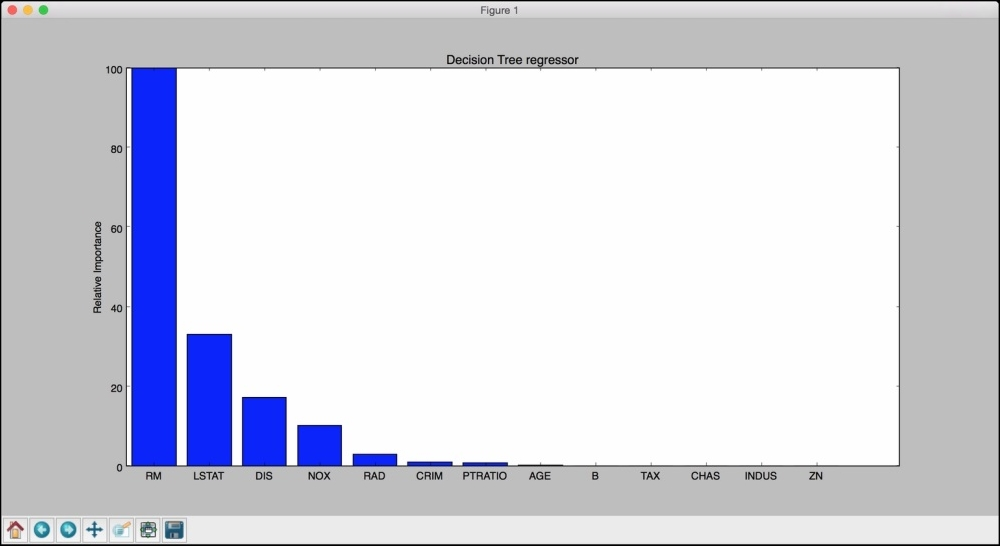4. So, the decision tree regressor says that the most important feature is RM. Let's take a look at what AdaBoost has to say in the following figure: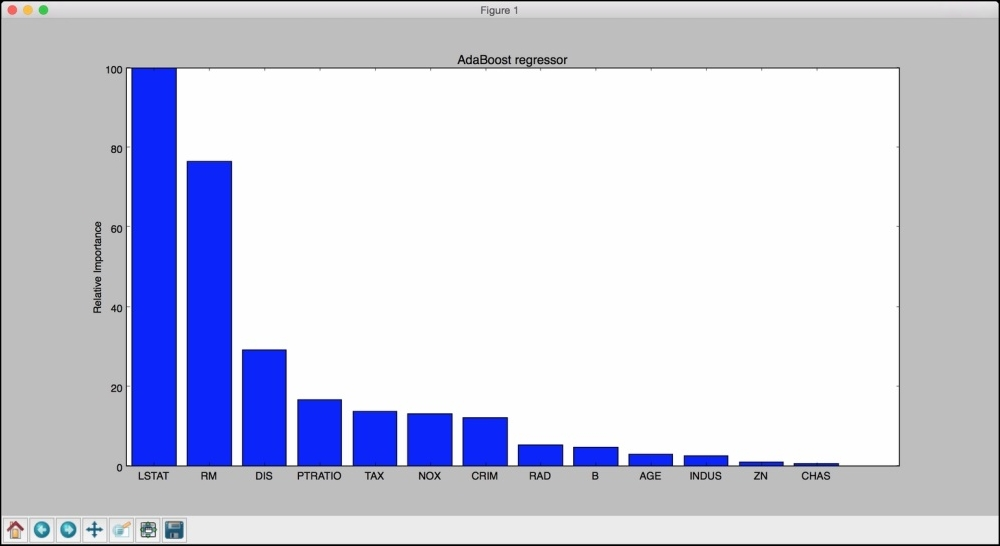According to AdaBoost, the most important feature is LSTAT. In reality, if you build various regressors on this data, you will see that the most important feature is in fact LSTAT. This shows the advantage of using AdaBoost with a decision tree-based regressor.

## Estimating bicycle demand distribution

Let's use a different regression method to solve the bicycle demand distribution problem. We will use the random forest regressor to estimate the output values. A random forest is a collection of decision trees. This basically uses a set of decision trees that are built using various subsets of the dataset, and then it uses averaging to improve the overall performance.

We will use the `bike_day.csv` file that is provided to you. This is also available at https://archive.ics.uci.edu/ml/datasets/Bike+Sharing+Dataset. There are 16 columns in this dataset. The first two columns correspond to the serial number and the actual date, so we won't use them for our analysis. The last three columns correspond to different types of outputs. The last column is just the sum of the values in the fourteenth and fifteenth columns, so we can leave these two out when we build our model.

### How to do it…

Let's go ahead and see how to do this in Python. You have been provided with a file called `bike_sharing.py` that contains the full code. We will discuss the important parts of this, as follows:

1. We first need to import a couple of new packages, as follows:

```import csv
from sklearn.ensemble import RandomForestRegressor
from housing import plot_feature_importances```
2. We are processing a CSV file, so the CSV package is useful in handling these files. As it's a new dataset, we will have to define our own dataset loading function:

```def load_dataset(filename):
X, y = [], []
X.append(row[2:13])
y.append(row[-1])

# Extract feature names
feature_names = np.array(X)

# Remove the first row because they are feature names
return np.array(X[1:]).astype(np.float32), np.array(y[1:]).astype(np.float32), feature_names```

In this function, we just read all the data from the CSV file. The feature names are useful when we display it on a graph. We separate the data from the output values and return them.

3. Let's read the data and shuffle it to make it independent of the order in which the data is arranged in the file:

```X, y, feature_names = load_dataset(sys.argv)
X, y = shuffle(X, y, random_state=7)  ```
4. As we did earlier, we need to separate the data into training and testing. This time, let's use 90% of the data for training and the remaining 10% for testing:

```num_training = int(0.9 * len(X))
X_train, y_train = X[:num_training], y[:num_training]
X_test, y_test = X[num_training:], y[num_training:]```
5. Let's go ahead and train the regressor:

```rf_regressor = RandomForestRegressor(n_estimators=1000, max_depth=10, min_samples_split=1)
rf_regressor.fit(X_train, y_train)```

Here, `n_estimators` refers to the number of estimators, which is the number of decision trees that we want to use in our random forest. The `max_depth` parameter refers to the maximum depth of each tree, and the `min_samples_split` parameter refers to the number of data samples that are needed to split a node in the tree.

6. Let's evaluate performance of the random forest regressor:

```y_pred = rf_regressor.predict(X_test)
mse = mean_squared_error(y_test, y_pred)
evs = explained_variance_score(y_test, y_pred)
print "\n#### Random Forest regressor performance ####"
print "Mean squared error =", round(mse, 2)
print "Explained variance score =", round(evs, 2)```
7. As we already have the function to plot the `importances` feature, let's just call it directly:

`plot_feature_importances(rf_regressor.feature_importances_, 'Random Forest regressor', feature_names)`

Once you run this code, you will see the following graph: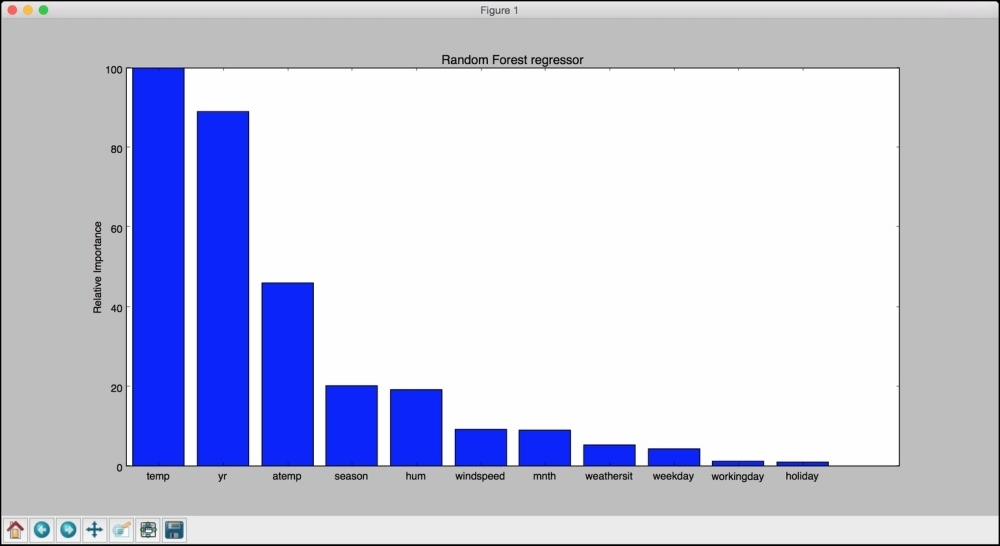Looks like the temperature is the most important factor controlling the bicycle rentals.

### There's more…

Let's see what happens when you include fourteenth and fifteenth columns in the dataset. In the feature importance graph, every feature other than these two has to go to zero. The reason is that the output can be obtained by simply summing up the fourteenth and fifteenth columns, so the algorithm doesn't need any other features to compute the output. In the `load_dataset` function, make the following change inside the for loop:

`X.append(row[2:15])`

If you plot the feature importance graph now, you will see the following: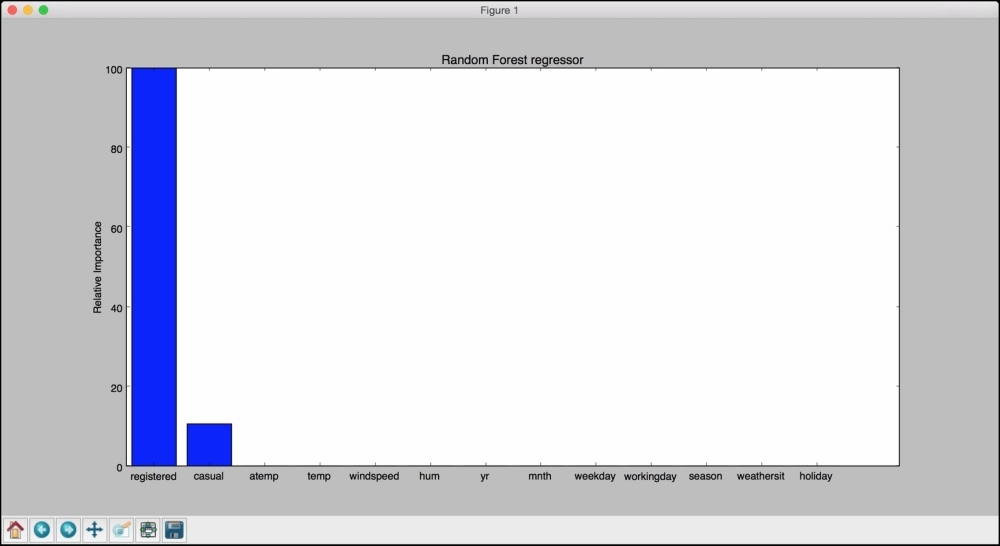As expected, it says that only these two features are important. This makes sense intuitively because the final output is a simple summation of these two features. So, there is a direct relationship between these two variables and the output value. Hence, the regressor says that it doesn't need any other variable to predict the output. This is an extremely useful tool to eliminate redundant variables in your dataset.

There is another file called `bike_hour.csv` that contains data about how the bicycles are shared hourly. We need to consider columns 3 to 14, so let's make this change inside the `load_dataset` function:

`X.append(row[2:14])`

If you run this, you will see the performance of the regressor displayed, as follows:

```#### Random Forest regressor performance ####
Mean squared error = 2619.87
Explained variance score = 0.92
```

The feature importance graph will look like the following: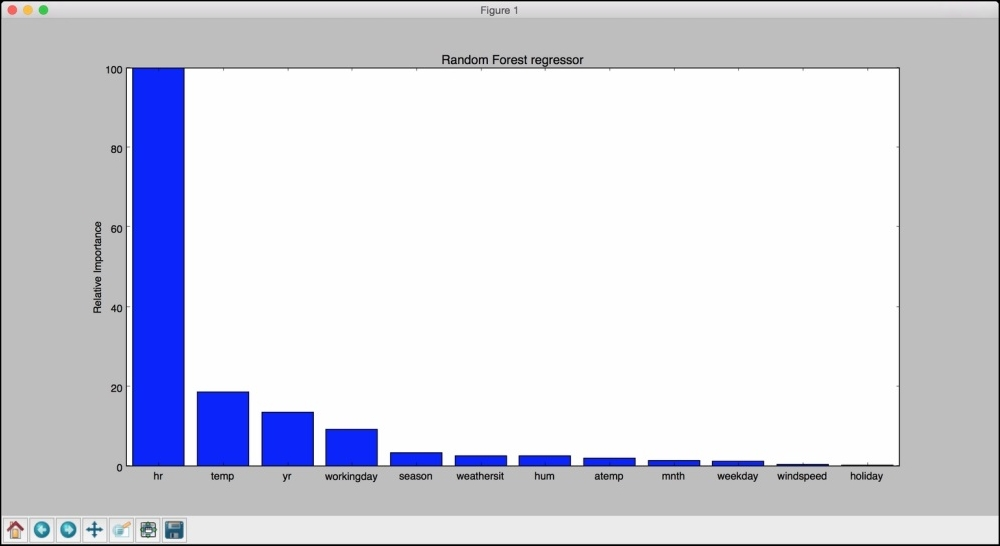This shows that the hour of the day is the most important feature, which makes sense intuitively if you think about it! The next important feature is temperature, which is consistent with our earlier analysis.